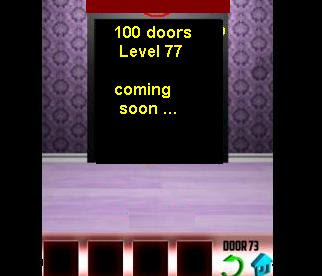100 Doors Level 77

The game is interesting because of the puzzle are not too easy to be beaten. So if you need 100 doors level 76 guide please follow the hints below"100 doors level 77" game

Welcome to math puzzle
the game ask you to add or to multiply
it's depend on how you can see the math operator
first you have to see number 1 x 5 then move to the right (remember the arrow is right direction) then you will find for number 2 the x become + now , so the equation is 2 + 8.

The complete answer for 100 doors 77 game is

• multiply 1 X 5 = 5
• add 2 + 6 = 8
• multiply 3 X 7 = 21
• add 8 + 4 = 12

so the code for this puzzle is 582112

We have updated our guide, hope this 100 doors level 77 solution can help you, for better understanding look at the image here